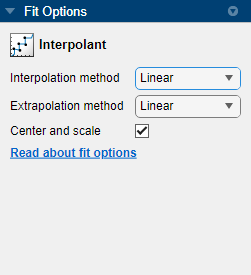Main Content

## Selecting an Interpolant Fit

### Selecting an Interpolant Fit Interactively

In the Curve Fitting app, select `Interpolant` from the model type list.

The `Interpolant` fit category fits an interpolating curve or surface that passes through every data point. For surfaces, the Interpolant fit type uses the MATLAB® `scatteredInterpolant` function for linear and nearest methods, the MATLAB `griddata` function for cubic and biharmonic methods, and the `tpaps` function for thin-plate spline interpolation.

The settings are shown here.You can specify the Method setting: ```Nearest neighbor```, `Linear`, `Cubic`, ```Shape-preserving (PCHIP)``` (for curves), `Biharmonic (v4)` (for surfaces) or `Thin-plate spline` (for surfaces). For details, see About Interpolation Methods.

Tip

If you are fitting a surface and your input variables have different scales, turn the Center and scale option on and off to see the difference in the surface fit. Normalizing the inputs can strongly influence the results of the triangle-based (i.e., piecewise `Linear` and `Cubic` interpolation) and `Nearest neighbor` surface interpolation methods.

For surfaces, try thin-plate splines when you require both smooth surface interpolation and good extrapolation properties.

### Fit Linear Interpolant Models Using the `fit` Function

This example shows how to use the `fit` function to fit linear interpolant models to data.

Interpolant Fitting Methods

Specify the interpolant model method when calling the fit function using one of the options outlined in Interpolant Model Names. None of the interpolant methods has any additional fit option parameters.

Fit a Linear Interpolant Model

Load data and fit a linear interpolant model using the `'linearinterp'` option.

```load census f = fit(cdate,pop,'linearinterp'); plot(f,cdate,pop);```Compare Linear Interpolant Models

Load data and create both nearest neighbor and pchip interpolant fits using the `'nearestinterp'` and `'pchip'` options.

```load carbon12alpha f1 = fit(angle,counts,'nearestinterp'); f2 = fit(angle,counts,'pchip');```

Compare the fitted curves `f1` and `f2` on a plot.

```p1 = plot(f1,angle,counts); xlim([min(angle),max(angle)]) hold on p2 = plot(f2,'b'); hold off legend([p1;p2],'Counts per Angle','Nearest Neighbor','pchip',... 'Location','northwest')```For an alternative to 'cubicinterp' or 'pchipinterp', you can use other spline functions that give you greater control over what you create. See About Splines in Curve Fitting Toolbox.

## Support

#### Machine Learning Challenges: Choosing the Best Classification Model and Avoiding Overfitting

Download white paper